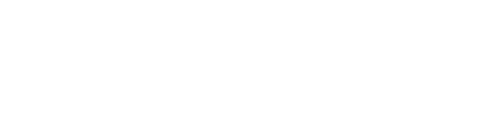# Classes and Objects C++ program to show the sum of an A.P. series

Write a c++ program to find out the sum of an A.P. series by using the classes and objects in object-oriented programming.

```#include<iostream>
using namespace std;
class T4Tutorials
{
protected :
int n1, df, n2, i, ln, s1;
public :
int apseries()
{
s1 = 0;
cout << "Find out the sum of A.P. series :"<<endl;
cout << "----"<<endl;
cout << "Input  the starting number of the A.P. series: "<<endl;
cin >> n1;
cout << "Input the number of items for  the A.P. series: "<<endl;
cin >> n2;
cout << "Input  the common difference of A.P. series: "<<endl;
cin >> df;
s1 = (n2 * (2 * n1 + (n2 - 1) * df)) / 2;
ln = n1 + (n2 - 1) * df;
cout << "The Sum of the  A.P. series are : " << endl;
for (i = n1; i <= ln; i = i + df)
{
if (i != ln)
cout << i << " + "<<endl;
else
cout << i << " = " << s1 << endl;
}
}

};
int main()
{
T4Tutorials a;
a.apseries();
}
```Output

Input the starting number: 2

Input the number of items: 4

Input the common difference: 6

the Sum of the A.P. series are :

2+8+14+20=44